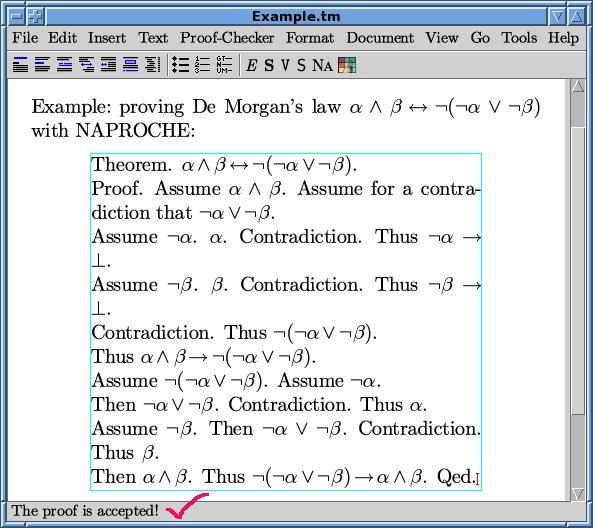The following example session with screenshots was carried out with an early NAPROCHE version programmed in SWI-Prolog, running under Suse-Linux and TeXmacs.

Start up the TeXmacs editor with the NAPROCHE plugin. The header line of TeXmacs will contain a "Proof-Checker" button. When pressed, it initiates proof checking of the current buffer.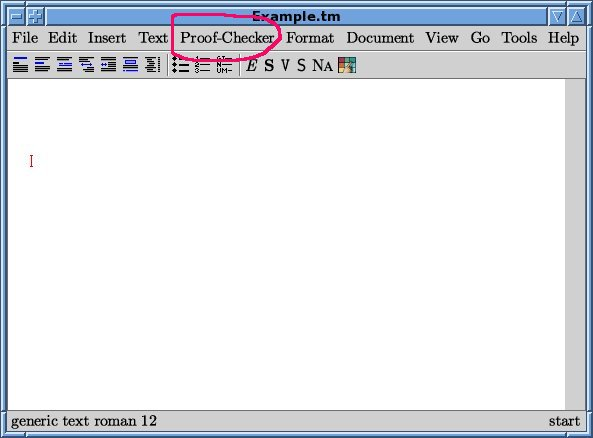Plain text editing with TeXmacs proceeds in the usual way. The mathematical mode is obtained by typing a \$-sign. Mathematical symbols like α can be entered by using the standard LaTeX commands and pressing <enter>. So ∖a l p h a and <enter> produces an α.A logical "and" (∧) can typed as ∖w e d g e or obtained with one mouse-click from the symbol menues provided in the mathematical mode, in this case from the menue denoted by ℘ .One can leave the mathematical mode by leaving the blue box around the mathematical text using the right-arrow keys (→) or by typing another \$-sign.

The text typed so far is treated as a comment by the proof-checker. Only text in the quotation environment is proof-checked. The quotation environment is available by typing <\quotation> (i.e., ∖q u o t a t i o n <enter>), or by using the menues Text > Environment > Quotation. Writing inside the quotation environment is possible just like outside.There are various editing tools like copying text by marking it with the mouse and pasting it by ctrl-y or by klicking the middle mouse button.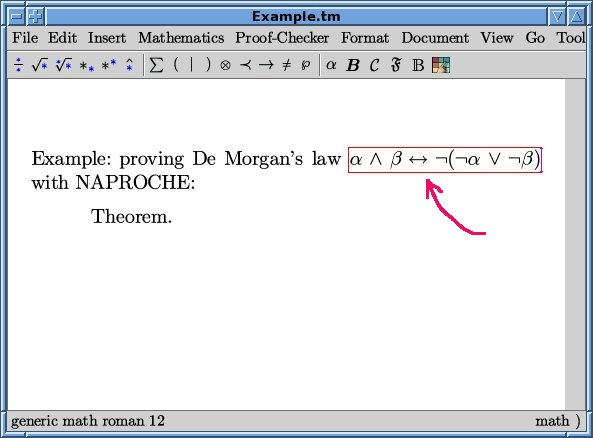The proof of the De Morgan equivalence proceeds in the standard way. Assume the left-hand side of the equivalence in order to obtain the right-hand side. A proof by contradiction may be initiated by "Assume for a contradiction that". Assumptions can be freely introduced in a proof. Note that the status line usually shows the internal state of the editor.The status line is also used by the NAPROCHE system for the two possible messages of the checker: "The proof is accepted" and "The proof is NOT accepted". So if one presses the Proof-Checker button now, the proof will be accepted, since the proof so far only consisted of the introduction of assumptions.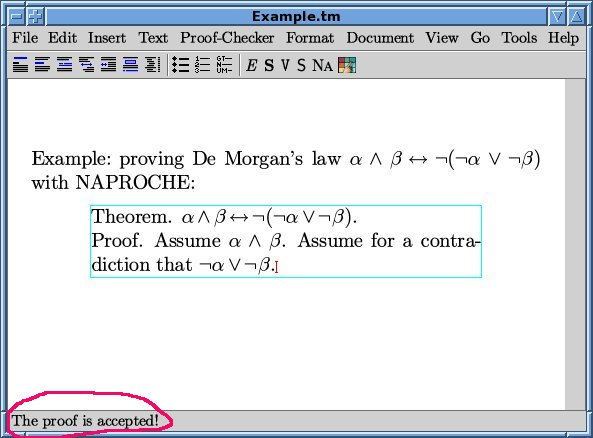If we introduce an (orthographical) mistake into the proof like starting a sentence with a small letter, the proof checker rejects the proof.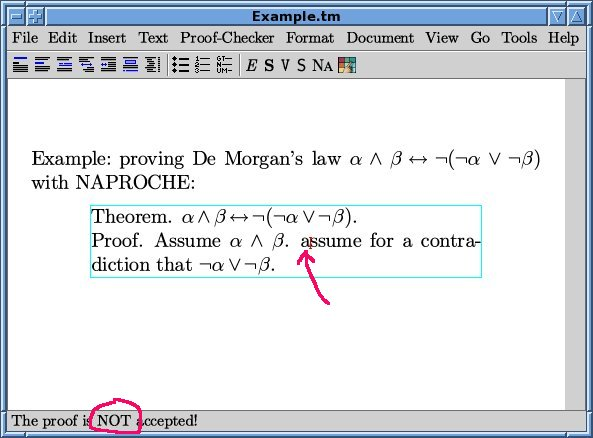So we correct the spelling mistake and continue the proof by assuming ¬α. Then α can be deduced from the assumption α∧β, and a contradiction can be deduced from the ¬α and α.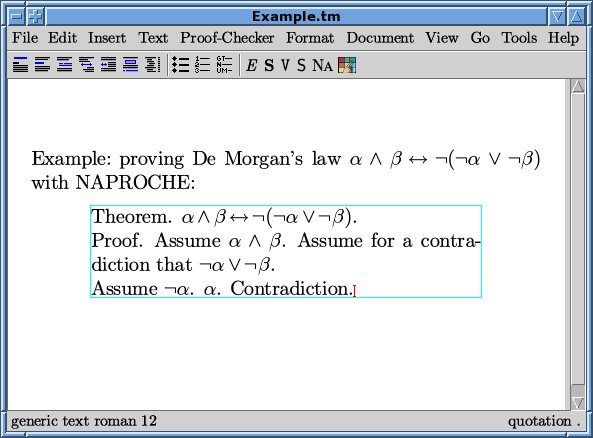This is vindicated by the proof-checker:This means that the last assumption implies a contradiction. This conclusion is expressed by the keyword "Thus":We proceed similarly for β:So each case of the disjunction ¬α∨¬β leads to a contradiction. So the converse ¬(¬α∨¬β) of the last open assumption holds. This consequence is also indicated by a "Thus".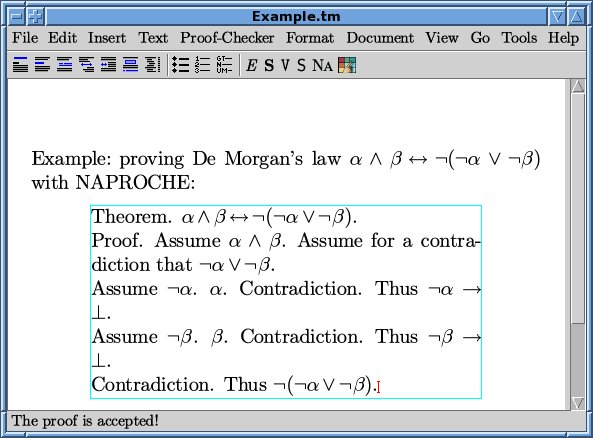Another application of "Thus" shows the left-to-right direction of the equivalence.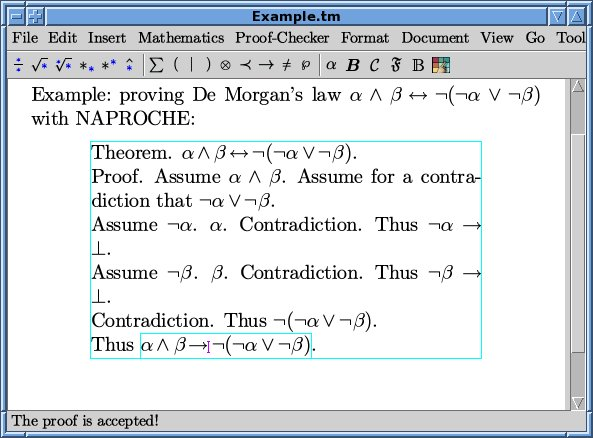A "Qed." requires checking the last open theorem against the statements established so far. Since the left-to-right implication does not imply the equivalence, the proof ended by "Qed." at this point is rejected.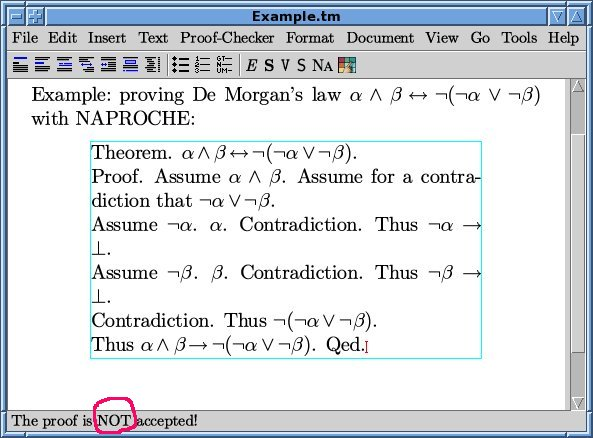Thus we remove the "Qed." and start the converse direction:To prove α∧β from this assumption, one assumes ¬α and uses rules of ∨-introduction and contradiction. Similarly one obtains β.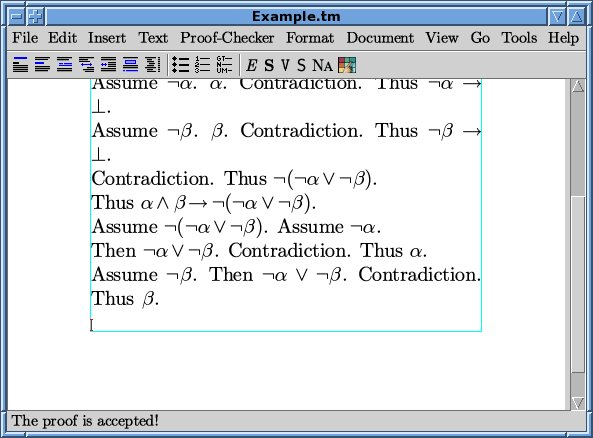α and β may be conjoined by ∧-introduction, and one gets the right-to-left implication.Now we can close the proof by "Qed." since → and ← imply ↔︎.